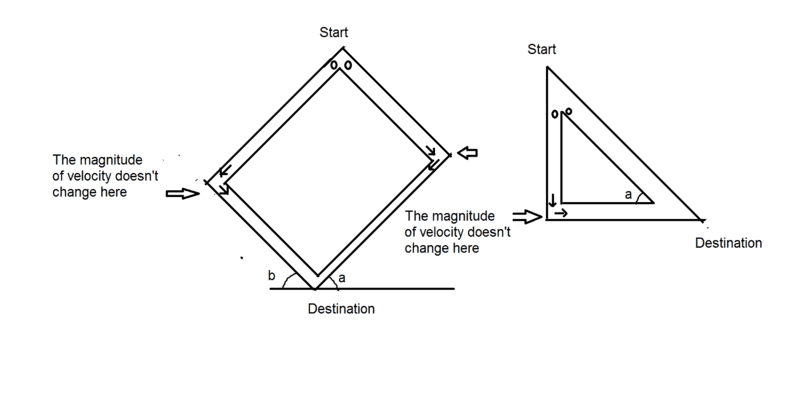# Time of Fall in triangular and rectangular frame

## Homework Statement

This question is actually two question. We have two hollow frames - one is rectangular and another is triangular. the rectangle is rotated and fixated such that the angles in shape are ##\alpha , \beta = 90 - \alpha## and the angle of triangle is ##\alpha##. We have two balls in each frame like the picture and we release them at the same time and when one gets to one end (except destination) it will continue its path with the same velocity magnitude and new direction so the velocity is never zero except for the start.

We want to find which ball gets to destination first. (Each frame is a different question and they are not related)

There is NO friction or air resistance but there is gravity (##g \approx 9.8 m/s^2##). Also the Thickness of frames are small and equal on each side and balls completely fit in the frame. The pictures are exaggerated. So no calculations needed for the Thickness. They both start with ##v = 0##.## The Attempt at a Solution

I tried to solve the triangle one, after finding speeds at end of the vertical path and solving the equations, and a lot of factorization and cancelling common terms out, I get something like ##cos \alpha / \sqrt{2 sin \alpha} + \sqrt{2 sin \alpha}## Compared to ## \sqrt{2 /sin \alpha}##. For the rectangular one, it gets a lot more complex. I want to know does it really depend to ##\alpha## and ##\beta##?

#### Attachments

Doc Al
Mentor
Not quite sure what you did there. For the triangle, I'd call the height h and find the times in terms of that variable (and the angle, of course). This should be a simple calculation. (Leave in terms of time squared, since all you want to do is see which gets there first.)

•titansarus
Not quite sure what you did there. For the triangle, I'd call the height h and find the times in terms of that variable (and the angle, of course). This should be a simple calculation. (Leave in terms of time squared, since all you want to do is see which gets there first.)
I called the hypotenuse ##d## And did this:
For the right one:
##1/2 ~g ~sin \alpha ~t^2 = d## so ## t = \sqrt{2d / (g~ sin\alpha)}##

For the left one:
##d ~sin\alpha = 1/2~~ g~ t_1^2 ## so ## t_1 = \sqrt{2d ~sin \alpha / g}##
the v at the bottom:

##2~g~d~sin\alpha = v^2## so ##v = \sqrt {2~g~d~ sin \alpha}##

now for time:

##v~ t_2 = d ~ cos \alpha## so ##t_2 = d ~cos\alpha / \sqrt{2~g~d~sin\alpha}##

Now for comparing between ##t## and ##t_1 + t_2## after factoring and canceling the equal terms, I get what I wrote above but I think It should be more simple. because the comparing between these two is not that simple.

Doc Al
Mentor
Oops, I missed a term in my own notes. Yes, it does look like a mess to compare. What I'd do is take the ratio of the times and play around with that.

Chandra Prayaga
I am missing something. In the triangle, i dont see why the ball falling virtically turns to the right at all. Will it not just bounce back up?

haruspex
Homework Helper
Gold Member
2020 Award
I am missing something. In the triangle, i dont see why the ball falling virtically turns to the right at all. Will it not just bounce back up?
We are told
it will continue its path with the same velocity magnitude and new direction
So they are to be thought of as smooth particles sliding in a track, presumably with little curves at the corners.

We are told

So they are to be thought of as smooth particles sliding in a track, presumably with little curves at the corners.
Yes. it is just the assumptions of the question. maybe there is little curve at the corners or some mechanism that change the direction of the velocity.

I am missing something. In the triangle, i dont see why the ball falling virtically turns to the right at all. Will it not just bounce back up?

It is just the assumptions of the question. It is not about an existing physical device. It is just a theoretical problem. You can think there is a tool there that change the speed direction very fast.

haruspex
Homework Helper
Gold Member
2020 Award
does it really depend to αα\alpha and ββ\beta?
It certainly depends on α for the triangle. Did you solve that?
For the rectangle, do we know anything about the relative lengths of the sides? E.g. is the start vertically above the finish?

Edit: it turns out the lengths don't matter.
To make the algebra easy, set g=1 and write s for sin(α), c for cos(α). Make up variables for the lengths.
Post your working as far as you get. It does simplify quite nicely.

Last edited:
It certainly depends on α for the triangle. Did you solve that?
For the rectangle, do we know anything about the relative lengths of the sides? E.g. is the start vertically above the finish?

I don't know how to solve that without any graphing calculator.

For the rectangle, We can just assume that the long side is named L and the short side W. and start is not above finish.

Unfortunately, the question has very few conditions. We just now the angle is ##\alpha , \beta## and the name of the sides.

haruspex
Homework Helper
Gold Member
2020 Award
I don't know how to solve that without any graphing calculator.
Write the equation for the two times being equal. This simplifies to a linear relationship between cos α and sin α.
There is a trick for solving such equations. Find an angle θ such that the equation can be written in the form sin(α+θ) = constant.

haruspex